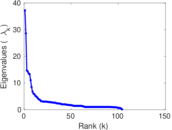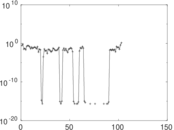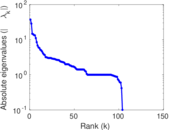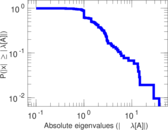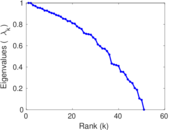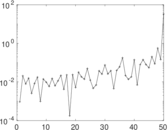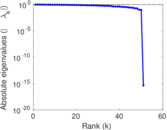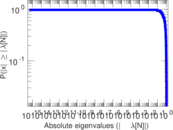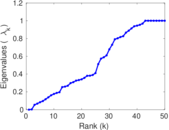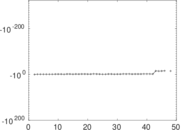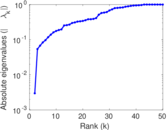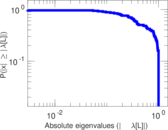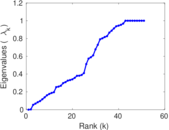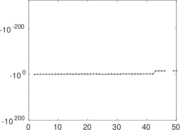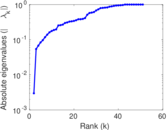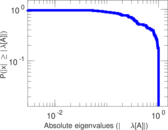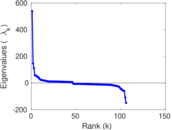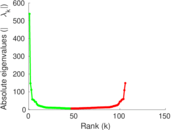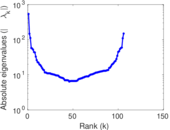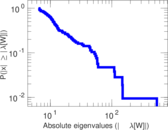# Wikiquote edits (zh-min-nan)

This is the bipartite edit network of the Chinese (Min Nan) Wikiquote. It contains users and pages from the Chinese (Min Nan) Wikiquote, connected by edit events. Each edge represents an edit. The dataset includes the timestamp of each edit.

 Code `qzh-min-nan` Internal name `edit-zh_min_nanwikiquote` Name Wikiquote edits (zh-min-nan) Data source http://dumps.wikimedia.org/ AvailabilityDataset is available for download Consistency checkDataset passed all tests Category Authorship network Dataset timestamp 2017-10-20 Node meaning User, article Edge meaning Edit Network formatBipartite, undirected Edge typeUnweighted, multiple edges Temporal dataEdges are annotated with timestamps

## Statistics

 Size n = 550 Left size n1 = 106 Right size n2 = 444 Volume m = 938 Unique edge count m̿ = 620 Wedge count s = 16,481 Claw count z = 626,009 Cross count x = 20,981,706 Square count q = 1,073 4-Tour count T4 = 75,808 Maximum degree dmax = 160 Maximum left degree d1max = 160 Maximum right degree d2max = 37 Average degree d = 3.410 91 Average left degree d1 = 8.849 06 Average right degree d2 = 2.112 61 Fill p = 0.013 173 6 Average edge multiplicity m̃ = 1.512 90 Size of LCC N = 375 Diameter δ = 16 50-Percentile effective diameter δ0.5 = 6.588 28 90-Percentile effective diameter δ0.9 = 8.899 46 Median distance δM = 7 Mean distance δm = 6.020 21 Gini coefficient G = 0.677 392 Balanced inequality ratio P = 0.220 682 Left balanced inequality ratio P1 = 0.202 559 Right balanced inequality ratio P2 = 0.318 763 Relative edge distribution entropy Her = 0.850 177 Power law exponent γ = 4.188 69 Tail power law exponent γt = 2.431 00 Tail power law exponent with p γ3 = 2.431 00 p-value p = 0.000 00 Left tail power law exponent with p γ3,1 = 1.741 00 Left p-value p1 = 0.084 000 0 Right tail power law exponent with p γ3,2 = 2.931 00 Right p-value p2 = 0.023 000 0 Degree assortativity ρ = −0.308 152 Degree assortativity p-value pρ = 4.185 98 × 10−15 Spectral norm α = 37.229 0 Algebraic connectivity a = 0.002 972 10 Spectral separation |λ1[A] / λ2[A]| = 1.302 15 Controllability C = 342 Relative controllability Cr = 0.621 818

## Plots

### Fruchterman–Reingold graph drawing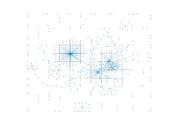### Degree distribution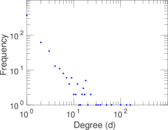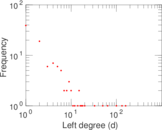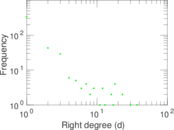### Cumulative degree distribution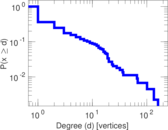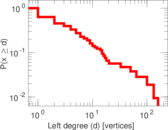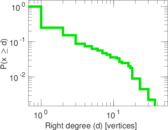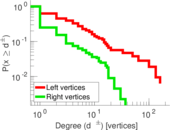### Lorenz curve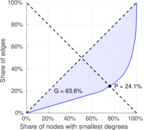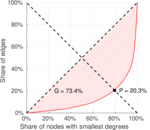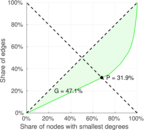### Spectral distribution of the adjacency matrix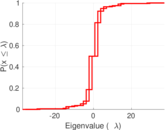### Spectral distribution of the normalized adjacency matrix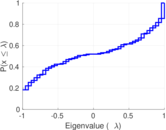### Spectral distribution of the Laplacian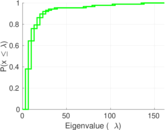### Spectral graph drawing based on the adjacency matrix### Spectral graph drawing based on the Laplacian### Spectral graph drawing based on the normalized adjacency matrix### Degree assortativity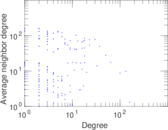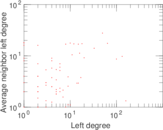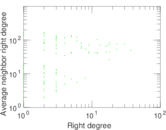### Zipf plot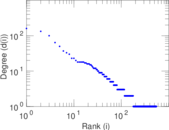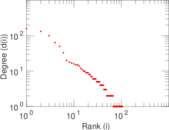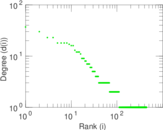### Hop distribution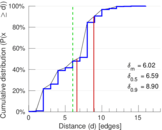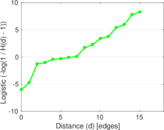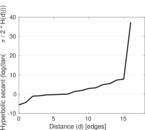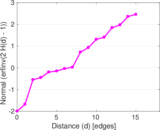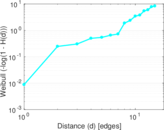### Double Laplacian graph drawing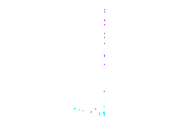### Delaunay graph drawing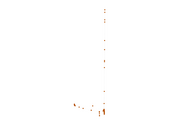### Edge weight/multiplicity distribution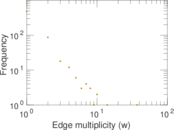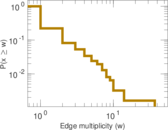### Temporal distribution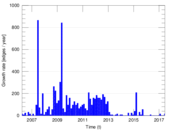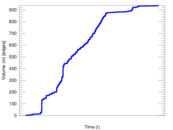### Temporal hop distribution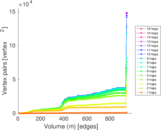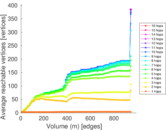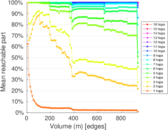### Diameter/density evolution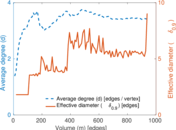### Matrix decompositions plots